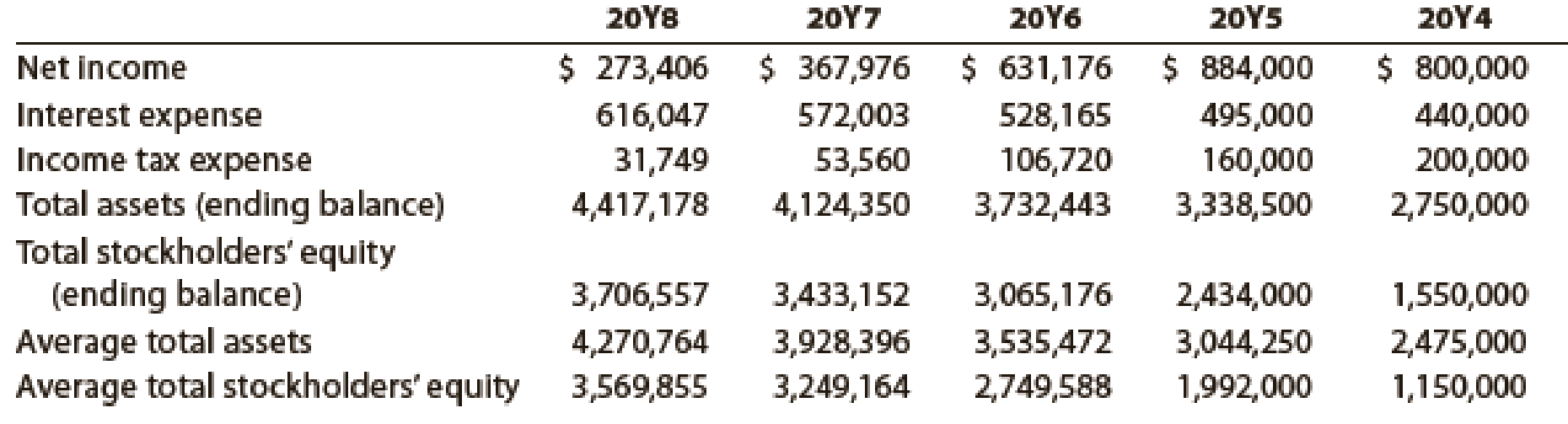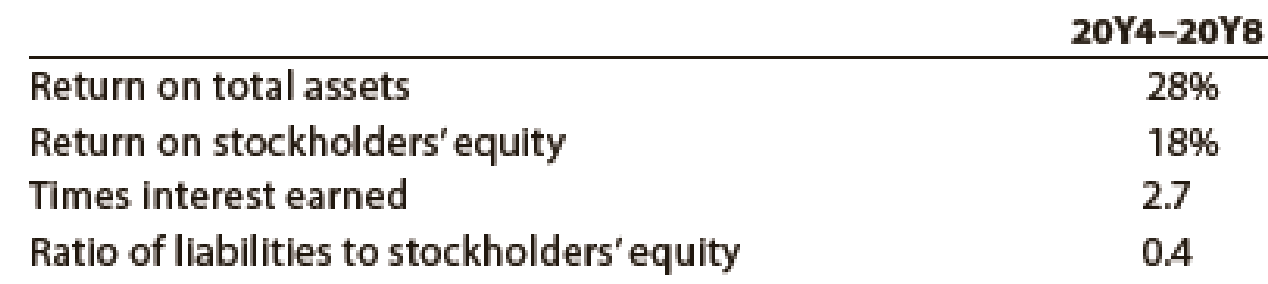Chapter 17, Problem 5PA

Chapter
Section
Textbook Problem
13 views

# Solvency and profitability trend analysisAddai Company has provided the following comparative information:You have been asked to evaluate the historical performance of the company over the last five years.Selected industry- ratios have remained relatively steady at the following levels for the last five years:Instructions 1. Prepare four line graphs with the ratio on the vertical axis and the years on the horizontal axis for the following four ratios, rounding to one decimal place: a. Return on total assets b. Return on stockholders’ equity c. Times interest earned d. Ratio of liabilities to stockholders’ equity Display both the company ratio and the industry benchmark on each graph. That is, each graph should have two lines. 2. Prepare an analysis of the graphs in (1).

1.

To determine

Determine the following ratios for five years:

1. (a) Return on total assets
2. (b) Return on stockholders’ equity
3. (c) Times interest earned ratio
4. (d) Ratio of total liabilities to stockholders’ equity

Also, display the determined ratios in a graph.

Explanation

Financial Ratios: Financial ratios are the metrics used to evaluate the liquidity, capabilities, profitability, and overall performance of a company.

a. Return on total assets for five years (20Y4 to 20Y8)

Rate of return on assets(20Y8)=Netincome + Interest expenseAverage total assets=$889,453$4,270,764=20.8%

Rate of return on assets(20Y7)=Netincome + Interest expenseAverage total assets=$939,979$3,928,396=23.9%

Rate of return on assets(20Y6)=Netincome + Interest expenseAverage total assets=$1,159,341$3,535,472=32.8%

Rate of return on assets(20Y5)=Netincome + Interest expenseAverage total assets=$1,379,000$3,044,250=45.3%

Rate of return on assets(20Y5)=Netincome + Interest expenseAverage total assets=$1,240,000$2,475,000=50.1%

Description:

Return on assets determines the particular company’s overall earning power. It is determined by dividing sum of net income and interest expense and average total assets.

Formula:

Rate of return on assets=Netincome + Interest expenseAverage total assets

b.

Return on stockholders’ equity for five years.

Rate of return on stockholders' equity(20Y8)}= Net income Average stockholder’s equity=$273,406$3,569,855=7.7%

Rate of return on stockholders' equity(20Y7)}= Net income Average stockholder’s equity=$367,976$3,249,164=11.3%Rate of return on stockholders' equity(20Y6)}= Net income Average stockholder’s equity=$631,176$2,749,588=23.0%Rate of return on stockholders' equity(20Y5)}= Net income Average stockholder’s equity=$884,000$1,992,000=44.4%

Rate of return on stockholders' equity(20Y4)}= Net income Average stockholder’s equity=$800,000$1,150,000=69.6%

Description:

Rate of return on stockholders’ equity is used to determine the relationship between the net income and the average common equity that are invested in the company.

Formula: Rate of return on stockholders' equtiy = Net incomeAverage  stockholder’s equity

c.

Times interest earned ratio for five years

Times-interest-earned ratio (20Y8) }=Net Income+Incometaxexpense+Interest expenseInterest expense=$921,202$616,047=1

2.

To determine

Prepare analysis of graphs.

### Still sussing out bartleby?

Check out a sample textbook solution.

See a sample solution

#### The Solution to Your Study Problems

Bartleby provides explanations to thousands of textbook problems written by our experts, many with advanced degrees!

Get Started

#### CASH BUDGETING Rework Problem 15-10 using a spreadsheet model. After completing Parts a through d, respond to t...

Fundamentals of Financial Management, Concise Edition (with Thomson ONE - Business School Edition, 1 term (6 months) Printed Access Card) (MindTap Course List)

#### Why is productivity important?

Principles of Macroeconomics (MindTap Course List)

#### Why will global sourcing increase?